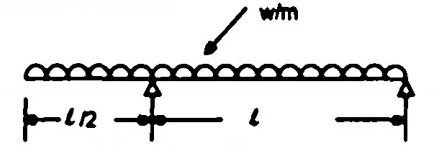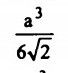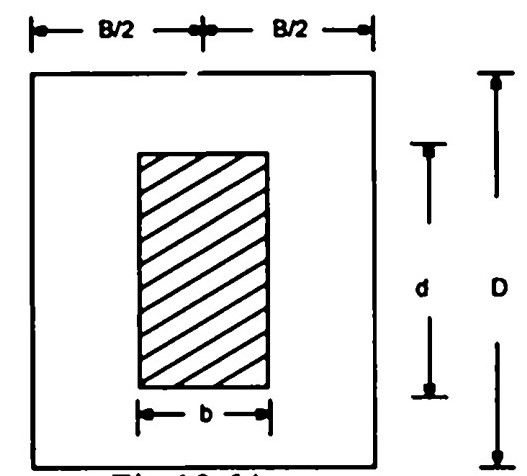top of pageSearch

# Strength of Materials(281-300)

Updated: Apr 29, 2020

281. A simply supported beam of constant width and varying depth and uniform

strength is subjected to a central concentrated load. The depth of the beam

dx at a distance x from one of the supports is proportional to x¹/²

282. A mild steel flat of width 120 mm and thickness 10 mm is bent into an arc of a

circle of radius 10 m by applying a pure moment 'M' If E is 2 x 10 N/mm², then

the magnitude of the pure moment 'M' will be 2xl0N-mm

283. The cross section of a beam is shown in Fig. (a). Its Iₓₓ is equal to 3 x 106 mm. It is subjected to a load as shown in Fig. (b)The maximum tensile stress in the beam would be

21 MN/m²

284.The shear stress distribution shown in Fig represents a beam with cross

section.285.The three-hinged arch shown in Fig. 10.60 will have value of H as

40 KN

286.287. A shaft turns at 150 rpm under a torque of 1500 Nm. Power transmitted is

7.5 πkW

288. A hollow steel shaft of external diameter 100 mm and internal diameter 50 mm is to be replaced by a solid alloy shaft. Assuming the same value of polar

modulus for both, the diameter of the solid alloy shaft will be289. A shaft of diameter 'd' is subjected to bending moment 'M' and twisting

moment 'T' The developed principal stress will be290.For the compound bar shown in Fig. 10.61, the ratio of stresses in the portions

AB: BC: CD will be 1:4:2

291.The ratio of total elongation of a bar of uniform cross-section produced under its own weight to the elongation produced by an external load equal to the weight of the bar is 1/2

292293.Figure shows a simply-supported beam overhanging to the left. The beam carries a uniformly distributed load of w/m throughout.The correct bending moment diagram for the beam is294. A beam of square section 'a' is used with its diagonal horizontal . The modulus of section of the beam is given by295.A section of a flitched beam is shown in

FigThe main role of the steel plate placed between the two timber sections is to

increase shear and bending resistance of the section

296. A three-hinged symmetric parabolic arch is hinged at the springings and at the crown. The span and rise are 40 m and 10 m respectively. The left half of the arch is loaded with U.D.L. of 3 t/m. The horizontal thrust at the springings will be

30 t

297.In the truss shown in Fig. 10.65 the forces in the members AB and BC will be

respectively (plus denotes tension) zero and zero

298. The deflection at the free end of a cantilever of rectangular cross-section due

to certain loading is 0.8 cm. If the depth of the section is doubled keeping the

width the same, then the deflection at the free end due to the same loading will be 0.1 cm

299. The ratio of torsional moments of resistance of a solid circular shaft of

diameter D and a hollow shaft having external diameter D and internal diameter

'd' is given by300.Two closely-coiled springs A and B of the same material, same wire and same

number of turns are subjected to an axial load of W. The mean diameter of spring A is half that of spring B. The ratio of deflection on spring B to that of spring A

will be 8